# 0708-1300/the unit sphere in a Hilbert space is contractible

Let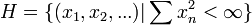$H=\{(x_1,x_2,...)| \sum x_n^2<\infty\}$ and define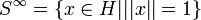$S^{\infty}=\{x\in H| ||x||=1\}$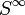$S^{\infty}$ is contractible
A way to see this is via the cellular structure of$S^{\infty}$. If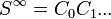$S^{\infty}=C_0 C_1 ...$ you can always contract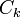$C_k$ along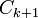$C_{k+1}$ like moving contracting the equator along the surface of the earth.